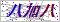PROUCTS LIST

NEWS

## 689=132=024电磁阀的详细资料：

Z689=132=024  电磁阀Z-689=132=024电磁阀配套与9245钠表使用。

9245-9240内部通讯协议模块    09200=A=5510
9245-9240的电势测量模块      09240=A=1500
9245-9240 钠离子分析仪的参比电极     09240=C=0310
9245-9240 钠离子分析仪的玻璃电极     09240=C=0320
DIPA 瓶，配有多孔渗水模块           09240=A=0510
KCl 3M  参比电极的电解液, 500 ml     363140,00500
PTFE 管路 ø 0.8X1.6mm （每米）     151065,08699
Tygon 管路ø 1.6X3.2mm （每米）     151399,90002
PTFE 管路 ø 2X6mm （每米）        151065,08699
PolyEthylene 管路 ø 4X6mm （每米）    151575,00006

ø 8mm O.D. 管路的快速配置    578=602=703
ø 6mm O.D. 管路的快速配置   578=601=703

9245钠表插入式过滤器       595=000=002
9245的电阀的连接器 （IP65）   09240=A=9170

9245钠表CPU板带显示屏     09200=A=1045

 如果你对689=132=024电磁阀感兴趣，想了解更详细的产品信息，填写下表直接与厂家联系：

### 留言框

• #### 验证码：请输入计算结果（填写阿拉伯数字），如：三加四=7QQ在线客服

• 15910526064